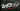# Web 3.0 ... or why we built the wrong internetself.__wrap_b=(o,n,e)=>{e=e||document.querySelector(`[data-br="\${o}"]`);let c=e.parentElement,a=h=>e.style.maxWidth=h+"px";e.style.maxWidth="";let t=c.clientWidth,l=c.clientHeight,s=t/2,i=t,d;if(t){for(;s+1<i;)d=~~((s+i)/2),a(d),c.clientHeight===l?i=d:s=d;a(i*n+t*(1-n))}e.__wrap_o||(e.__wrap_o=new ResizeObserver(()=>{self.__wrap_b(0,+e.dataset.brr,e)})).observe(c)};self.__wrap_b(":Rll:",1)

blog
react
web
blockchain
crypto